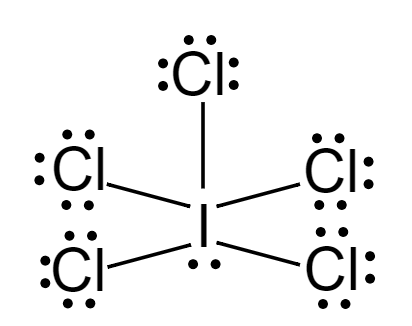Courses
Courses for Kids
Free study material
Offline Centres
MoreLast updated date: 08th Dec 2023
Total views: 281.7k
Views today: 3.81k

# What atomic or hybrid orbitals make up the sigma bond between $I$ and $Cl$ in iodine pentachloride, $IC{l_5}$?Verified
281.7k+ views
Hint: The intermixing of two or more pure atomic orbitals of an atom with almost the same energy to give the same number of identical and degenerate new types of orbitals is known as hybridization. The new orbitals formed are also known as hybrid orbitals.
Formula to find the hybridization of a molecule is given by
$Hybridisation = Number{\text{ }}of{\text{ }}\sigma {\text{ }}bonds + {\text{ }}Number{\text{ }}of{\text{ }}lone{\text{ }}pairs$

If the sum is $2$ $\to$ hybridization − $sp$
If the sum is $3$ $\to$ hybridization − $s{p^2}$
If the sum is $4$ $\to$ hybridization − $s{p^3}$
If the sum is $5$ $\to$ hybridization− $s{p^3}d$
If the sum is $6$ $\to$ hybridization− $s{p^3}{d^2}$
Lewis dot structure of $IC{l_5}$:Considering central metal atom, $I$
$Number{\text{ }}of{\text{ }}lone{\text{ }}pairs = 1 \\ Number{\text{ }}of{\text{ }}bond{\text{ }}pairs = 5 \\$
$Hybridisation = 1 + 5 = 6$
Therefore, $I - Cl$ bond is $s{p^3}{d^2}$ hybridized.
About $IC{l_5}$,
Hybridisation-$s{p^3}{d^2}$
Geometry- Octahedral
Shape- Square pyramidal

Note:
You can expect bond angles of ${90^ \circ }$ and ${180^ \circ }$.
Also note that when forming hybrid orbitals, you use $n$ atomic orbitals to make $n$ hybrid orbitals, where $n$ is the total amount of orbitals. Here, in case of $IC{l_5}$, $n = 6$